# VLOOKUP formula across multiple sheets

September 28, 2021
507 Views

### Graphic skills

In the following example, Sheet1'Client Order List' records the equipment types ordered by corresponding client company names, while Sheet2 'Price List' lists Sequence No. and Price for each type of equipment.

Now we want to fill in Column D ' for each company, using the VLOOKUP function with data in Sheet2.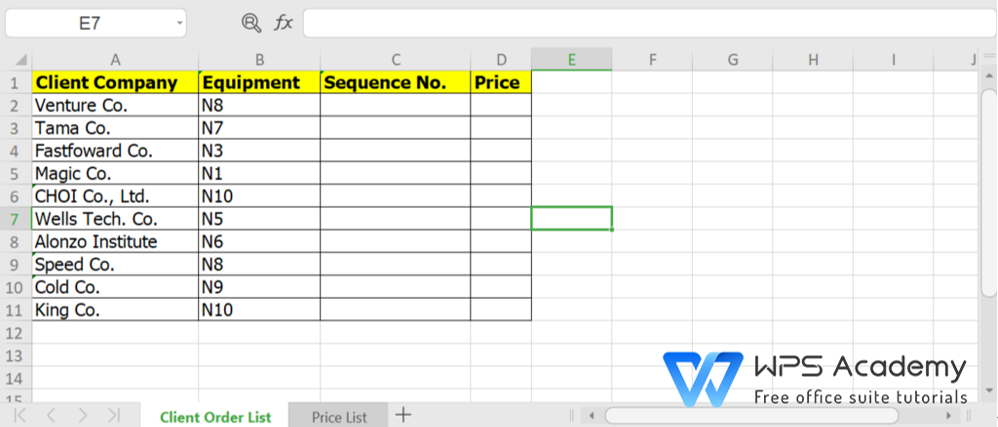Sheet1: Client Order List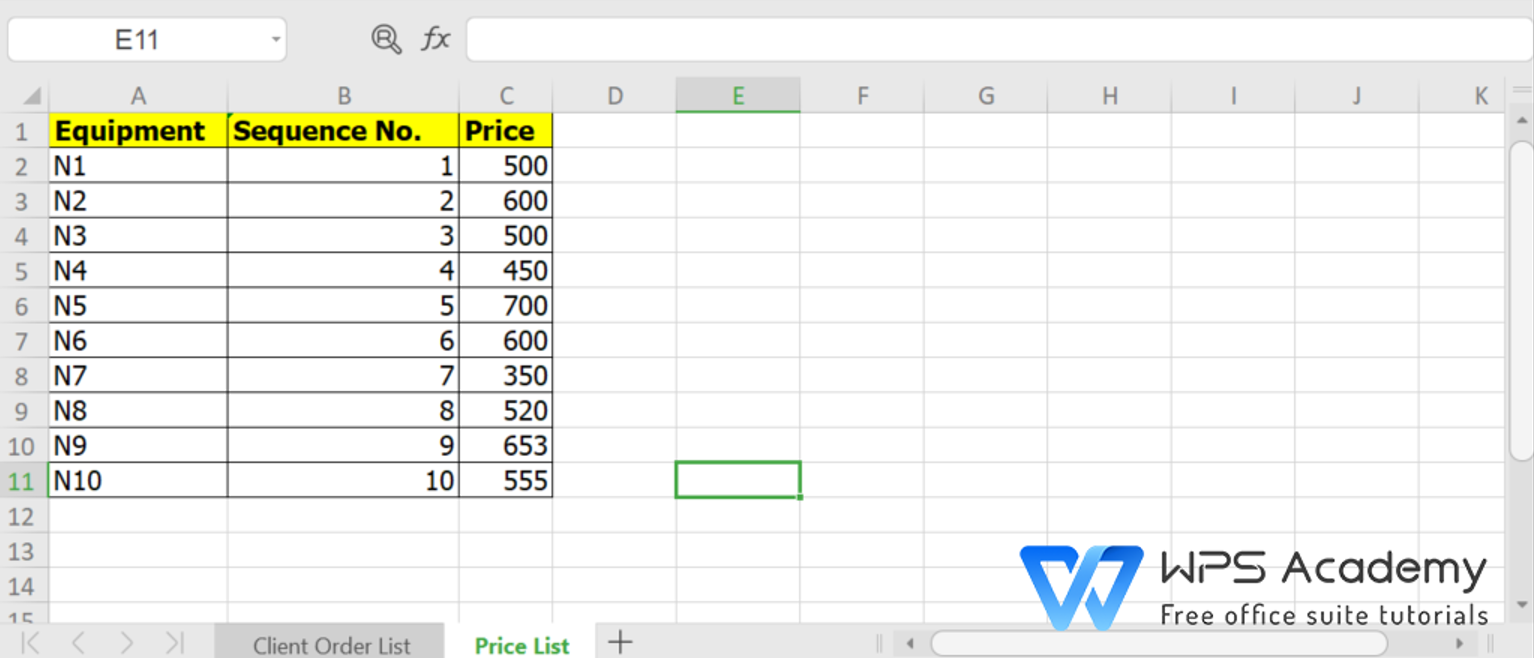Sheet2: Price List

· Option 1:

1. Input =VLOOKUP(B2,'Price List'!\$A\$1:\$C\$11,3,FALSE) in cell C2 of Sheet1 'Client Order List'.

2. Select cell D2, and drag the fill handle (+) to run the Auto Fill function.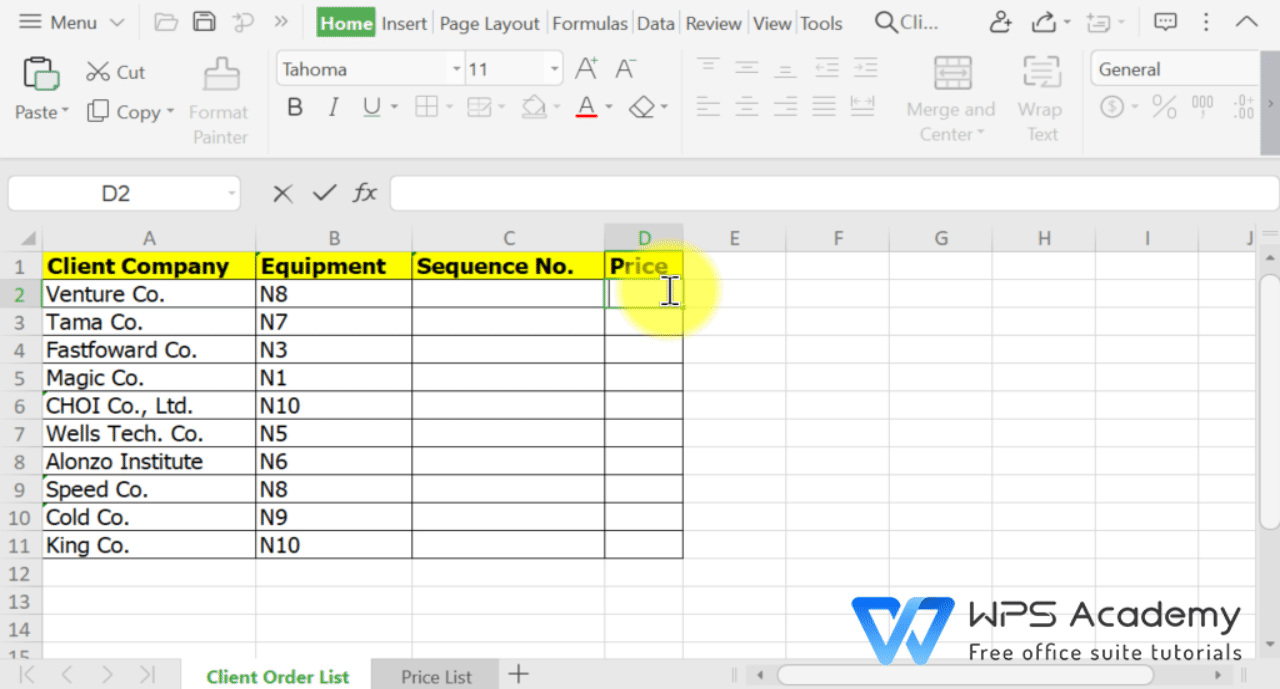· Option 2:

1. Input =VLOOKUP( in cell C2 of Sheet1 'Client Order List'.

2. Click or select the components included in the formula, with a comma inserted between each two factors.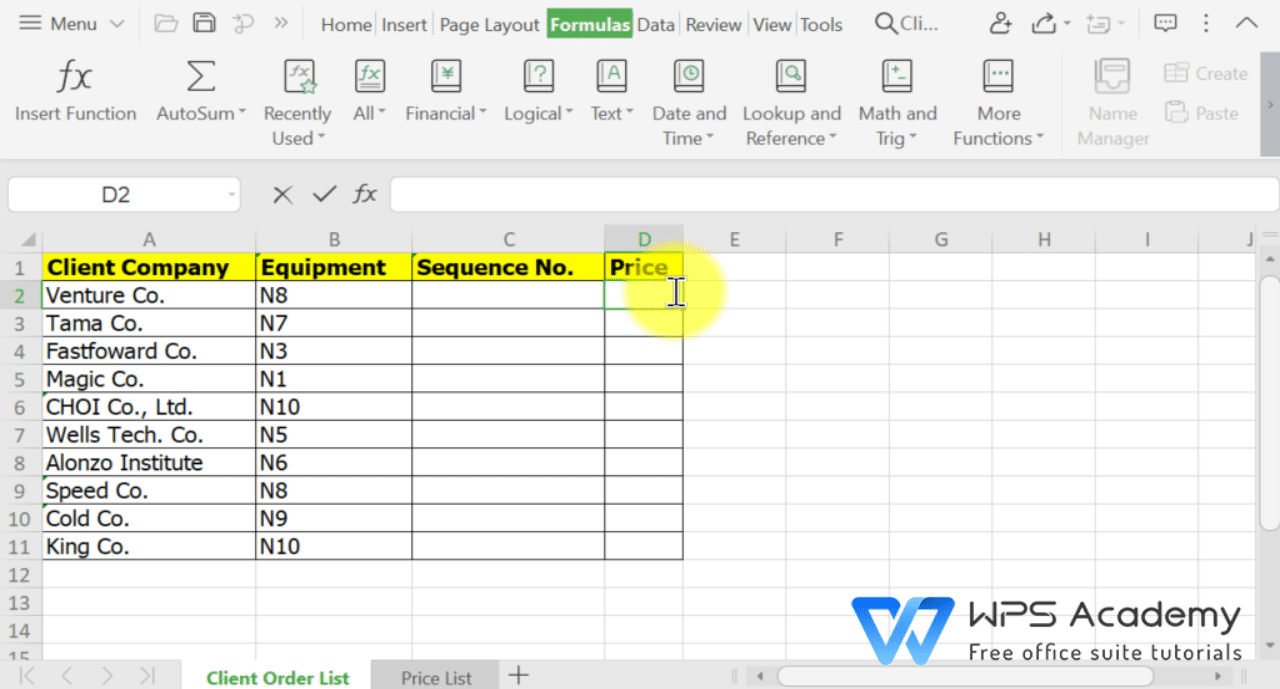3. Input '\$' in corresponding places to lock the selected lookup range.

4. Press Enter to run the formula.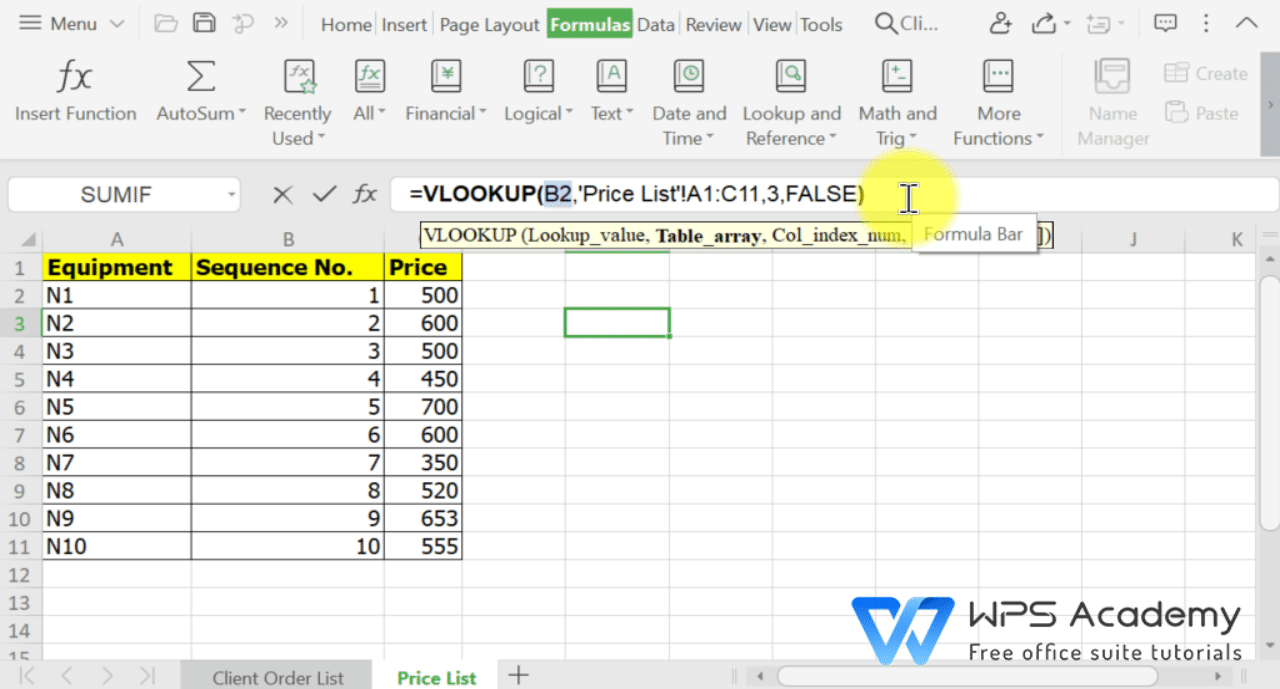5. Select D2, and drag the fill handle (+) to run the Auto Fill function.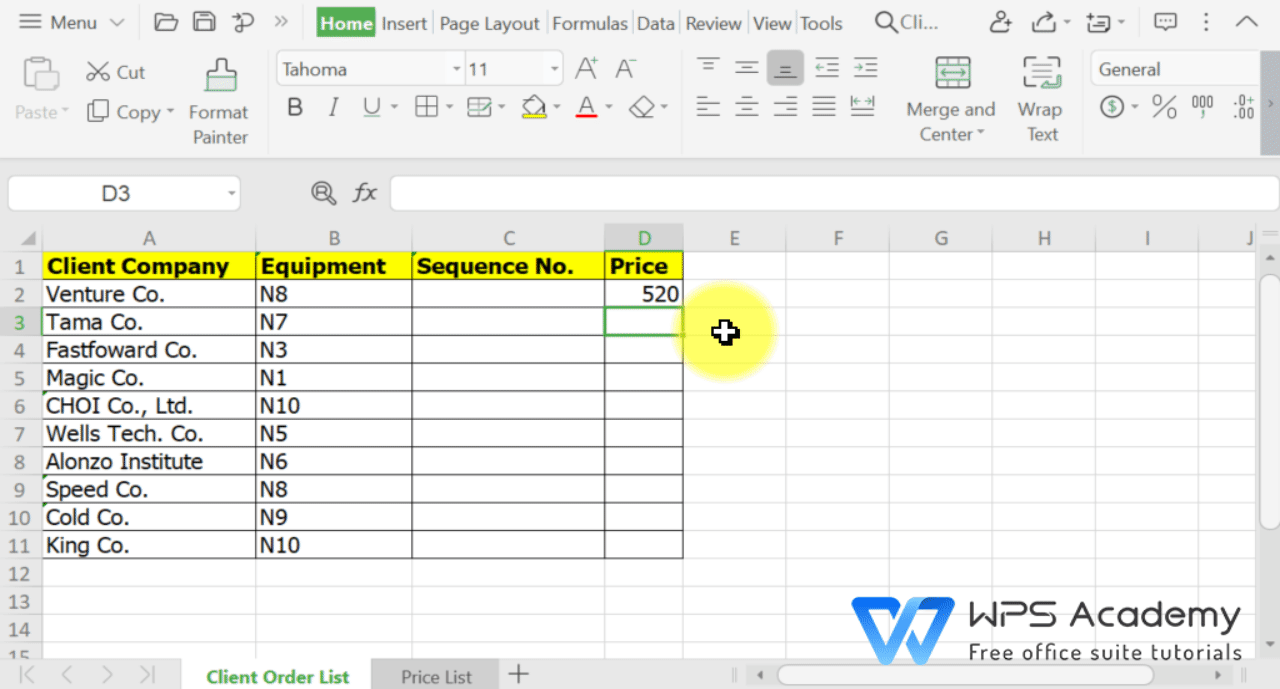· Notes:

VLOOKUP formula includes FOUR components.

Lookup_value: normally the values meanwhile involved in both sheets

Table_array: the range where to look up

Col_index_num: the order of the column where the data to be filled locates in

[Range_lookup]: FALSE (for exact match) / TRUE (for approximate match)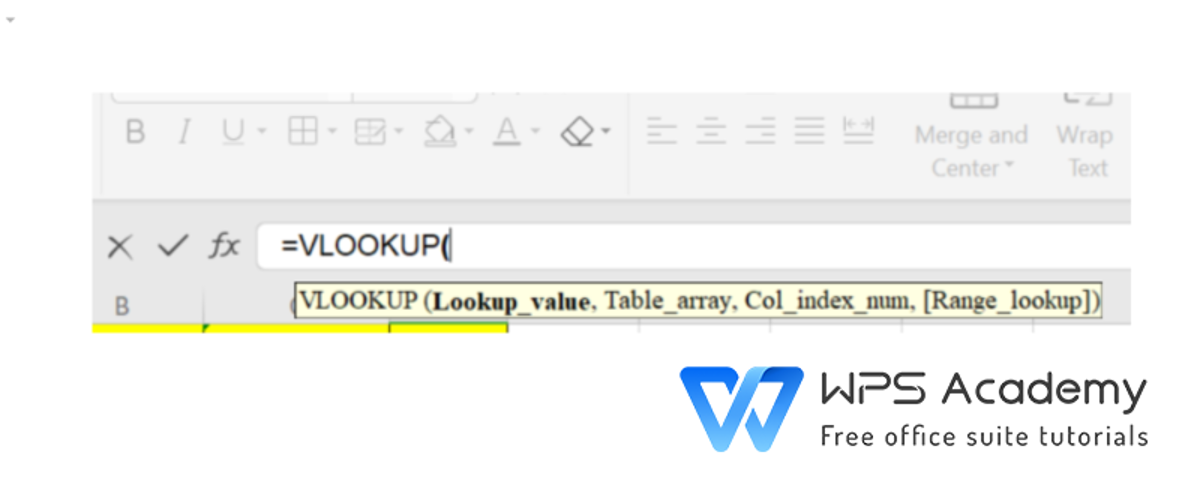VLOOKUP formula

· Here are the exact meanings of each factor involved in the formula.

 Component(s) of the formula Corresponding exact meanings (B2) the value to be matched, normally the values involved in the both sheets ('Price List'!\$A\$1:\$C\$11) the range to look up values is \$A\$1:\$C\$11 of the Sheet 'Price List' (3) the information to be filled in comes from the 3rd column in the selected range (FALSE) exact match

To be office excel advanced, you could learn how to use WPS Office Spreadsheet online in WPS Academy.